## Cost Benefit Formulation

To make the analysis simple, we will consider only one bus in one route of operation. All cost and benefit are computed for one year and then we convert them into present value for all the usage years. The formulations below are simplified version of our common sense. Below each formula is the unit check to make sure that the formulations are correct.

### Cost Formulation

We compute the total costs and total benefit for one year and assume the pricing to be the same for all the usage years (e.g. 5 years). We break down the operation and maintenance cost of the bus into gasoline, engine oil, tire and driver. For simplicity of the computation, we combine all the administrative cost and simple maintenance (such as cleaning, painting) into yearly administrative cost.(1)(2)(3)

Total driver cost per year is equal to the driver monthly salary and 12 months per year. (If the social regulation states that consider some 2 months extra bonus of the driver salary per year, then you can change 12 into 14).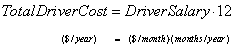(4)

Total operating cost per year is the summation of all costs above plus yearly administrative cost. The units are all in monetary terms (\$/year). Since each item is computed for one year, the total operating cost is also for one year. Note that the administrative cost is already lump sum for one year.(5)

Total cost must be converted into present value. The present total cost include the initial purchasing cost plus the yearly operating cost that converted into present value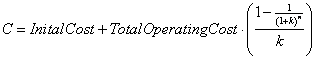(6)

Factor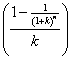is used to convert annuity into present value. The annuity starts from the end of this year (or beginning of first year). We will discuss more about this factor in the Financial Analysis.

### Benefit Formulation

To compute total benefit per year, first we compute daily benefit. Daily benefit is depending on the number of passenger per cycle and fare per passenger and number of cycle per day. The fare is constant per passenger per trip (not per cycle). Passengers pay per one ride. Since the maximum fare is set to be \$1, we will use this value. See the explanation below for the formulation and how to get the value of each factor.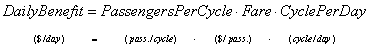(7)

The total benefit is the summation of daily benefit for one year and annuity of salvage value plus possible subsidy. Subsidy is only be given by the local government if the system is not feasible from the running benefit alone but feasible within the limit of maximum subsidy. If total benefit that has included maximum subsidy and still not feasible, then the system is really not feasible.(8)

Salvage value is only one time in the future. Thus, to make it into one year, we convert it into annuity using factor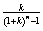.

Having all the benefit in yearly basis, now we can convert the total benefit into present time so that we can compare it with the total cost.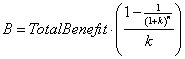(9)

Factoris used to convert annuity into present value. The annuity starts from the end of this year (or beginning of first year). We will discuss later about this conversion factors in the Financial Analysis.

### Bus Operation Formulation

Total distance is computed for one year. If we know the number of working day per year and total daily distance, then we can compute total distance as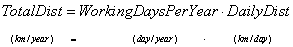(10)

Total daily distance is depending on the number of cycle per day and distance per cycle.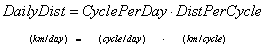(11)

Number of cycle per day is an integer of a ratio between number of working hours per day and time per cycle.(12)

Time per cycle is depending on the travel time (which is equal to cycle per km divided by the operating speed of the bus) plus some time reduction due stopping time to boarding and alighting of passengers along the way and some reduce time due to slowing down to get the passengers). Let us assume the effect of traffic congestion is already included in the vehicle operating speed for simplicity.(13)

In one cycle (from origin station to destination station and back to origin), total number of passengers may exceed vehicle capacity depending on the demand of every hour. They don't stay all the time inside the bus from origin station to destination. Some passengers may go in and go out during the travel inside the cycle. This fluctuation of demand is represented by simple constant name Load Factor. [Remember that in reality load factor is not constant but fluctuate over time. In here, we use the average load factor and assume it as constant]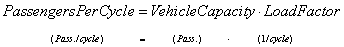(14)

Preferable reference for this tutorial is

Teknomo, Kardi (2006) Tutorial on Feasibility Study. http://people.revoledu.com/kardi/tutorial/What-If-Analysis/index.html